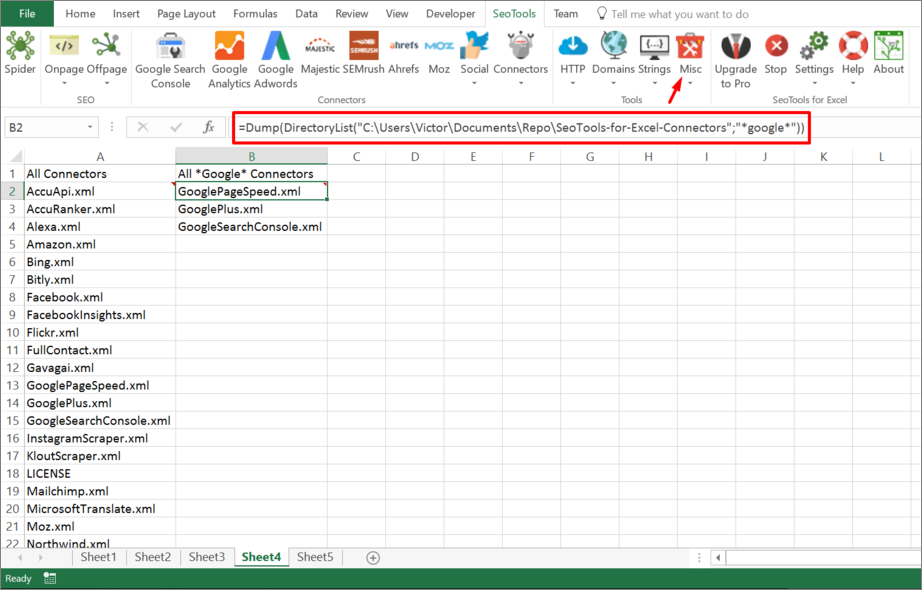``````=DirectoryList(
string path,
string filter (optional),
bool listDirectories (optional; default=FALSE),
bool absolutePaths (optional; default=FALSE),
bool recursive (optional; default=FALSE)
) : vector
``````## Purpose

Returns array with files, directory names or absolute paths.

### Filter

Apply this criteria to filter the results. In the following example (see picture above) I've filtered for files containing google

``````=Dump(DirectoryList("C:\ExamplePath\","*google*"))
``````

### ListDirectories

Apply this criteria (`True|False`) to return directories.

``````=Dump(DirectoryList("C:\ExamplePath\",,TRUE))
``````

### AbsolutePaths

Apply this criteria (`True|False`) to return the path of the matching results instead of the names.

``````=Dump(DirectoryList("C:\ExamplePath",,,TRUE))
``````

## Recursive

Apply this criteria (`True|False`) to return results from sub directories.

``````=Dump(DirectoryList("C:\ExamplePath",,,,TRUE))
``````

## Dump

Dump is a very useful function for working with functions that returns arrays.